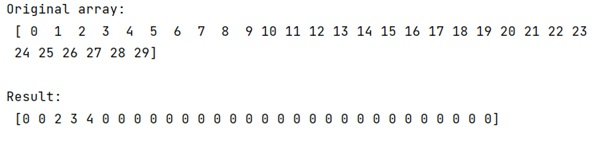# Change the values of a NumPy array that are NOT in a list of indices

Learn, how to change the values of a NumPy array that are NOT in a list of indices in Python? By Pranit Sharma Last updated : March 31, 2023

Suppose that we are given a numpy array and an array of indices and we need to change the values of this numpy array that are NOT in array of indices.

## NumPy Array - Change the values that are NOT in a list of indices

To change the values of a NumPy array that are NOT in a list of indices, we need to first create a numpy array and an array of indices and then we can use numpy.setxor1d() which will help us to find the exclusive OR operation between these two arrays. We will store the result of setxor1d() in a variable, now this result is an array of those values which are common in an array and index array. We will assign these values with another value.

Let us understand with the help of an example,

## Python code to change the values of a NumPy array that are NOT in a list of indices

```# Import numpy
import numpy as np

# Creating numpy array and index array
arr = np.arange(30)
index = [2, 3, 4]

# Display Original array
print("Original array:\n",arr,"\n")

# Changing values that are not in index
ix = np.indices(arr.shape)

not_in = np.setxor1d(ix, index)
arr[not_in] = 0

# Display result
print("Result:\n",arr,"\n")
```

### Output